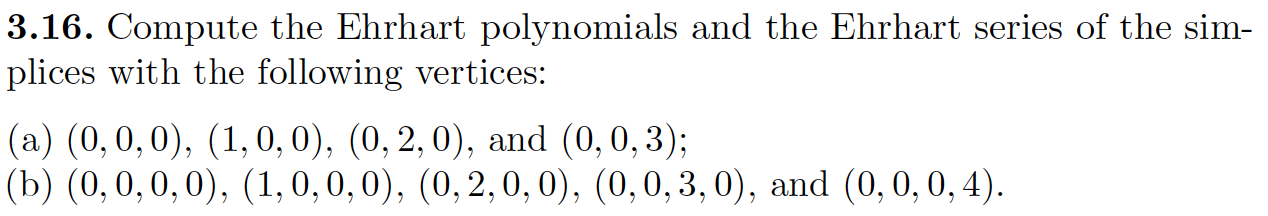### Create an Account

Home / Questions / 3.16. Compute the Ehrhart polynomials and the Ehrhart series of the sim- plices with the f...

# 3.16. Compute the Ehrhart polynomials and the Ehrhart series of the sim- plices with the following vertices: (a) (0,0,0), (1,0,0), (0,2,0), and (0,0,3); (b) (0,0,0,0), (1,0,0,0), (0,2,0,0), (0,0,3,0),

3.16. Compute the Ehrhart polynomials and the Ehrhart series of the sim- plices with the following vertices: (a) (0,0,0), (1,0,0), (0,2,0), and (0,0,3); (b) (0,0,0,0), (1,0,0,0), (0,2,0,0), (0,0,3,0), and (0,0,0,4).Apr 20 2021 View more View LessSubscribe To Get Solution